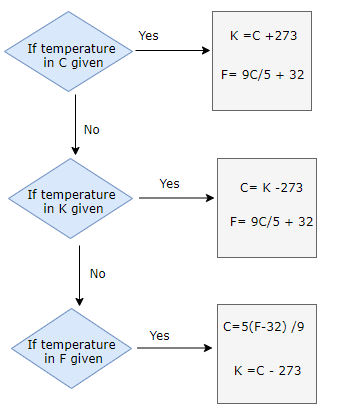# Temperature Conversion calculator

Note
• Enter the values of the one known variable in the text boxes
• Leave the text box empty for the variable you want to solve for
• Click on the calculate button.
The formula used for solving the question is
$\deg C= K -273$
$F -32 =\frac{9}{5}C$

## What is temperature and Temperature Scale

Temperature is the measurement of how hot or cold the body is. Temperature is measured using thermometer.Construction of thermometers generally requires a measurable property of a substance which monotonically changes with temperature.
We have three common scale of temperature
(a) Celsius Scale: Temperature in Celsius is measured in degree.It is denoted by ° C.Melting point of ice and boiling point of water have values 0 ° C and 100° C
(b) Fahrenheit Scale : Temperature in Celsius is measured in degree but it is smaller than celsius.It is denoted by ° F.Melting point of ice and boiling point of water have values 32 ° F and 132° F
(b) kelvin Scale : Size of a degree in Celsius and kelvin scale are sam.It is denoted by K.Melting point of ice and boiling point of water have values 273.15 K and 373.15 K
Relation Between Celsius ,Fahrenheit and Kelvin
$\frac {C -0}{100} = \frac {F -32}{180} =\frac {K - 273}{180}$

Example of Few questions where you can use this formula
Question 1
Convert 45 ° C to Kelvin and Fahrenhiet Scale
Solution
We have the relation
$\frac {C -0}{100} = \frac {F -32}{180} =\frac {K - 273}{180}$
Taking $\frac {C -0}{100} = \frac {F -32}{180}$
$F= \frac {9}{5}C + 32= \frac {9}{5} \times 45 + 32= 113$ ° F
Taking $\frac {C -0}{100} =\frac {K - 273}{180}$
$K= C + 273 = 45 + 273 = 318 \ K$

Question 2
Convert 23 K to ° C and Fahrenhiet Scale.
Solution
We have the relation
$\frac {C -0}{100} = \frac {F -32}{180} =\frac {K - 273}{180}$
Taking $\frac {C -0}{100} =\frac {K - 273}{180}$
$C= K - 273= 23 - 273= -250$ ° C
Taking $\frac {C -0}{100} = \frac {F -32}{180}$
$F= \frac {9}{5}C + 32= \frac {9}{5} \times (-250) + 32= -418$ ° F

## How the Temperature Conversion calculator works1. If Temperature in Celsius is given, Temperature in Kelvin and Fahrenhiet can be found using
$K= C + 273$
$F= \frac {9}{5}C + 32$
2. If Temperature in Kelvin is given, Temperature in Celsius and Fahrenhiet can be found using
$C= K - 273$
$F= \frac {9}{5}C + 32$
3. If Temperature in Fahrenhiet is given, Temperature in Celsius and Kelvin can be found using
$C = \frac {5(F-32)}{9}$
$K= C + 273$